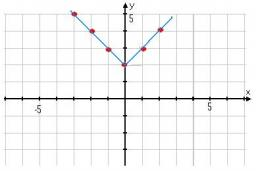# Original 4777

Which number has such a property that if we increase it by 7, we get a number with the same absolute value as the original number?

x =  -3.5

### Step-by-step explanation:

(x+7)=-x

2x = -7

x = -7/2 = -3.5

x = -7/2 = -3.5

Our simple equation calculator calculates it.Did you find an error or inaccuracy? Feel free to write us. Thank you!

Tips for related online calculators
Do you have a linear equation or system of equations and looking for its solution? Or do you have a quadratic equation?